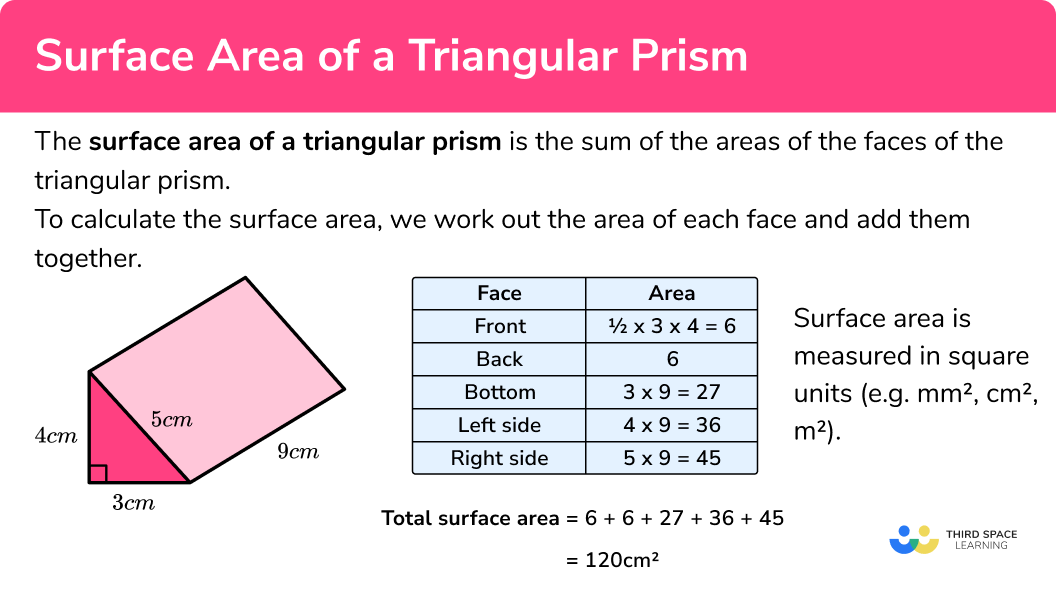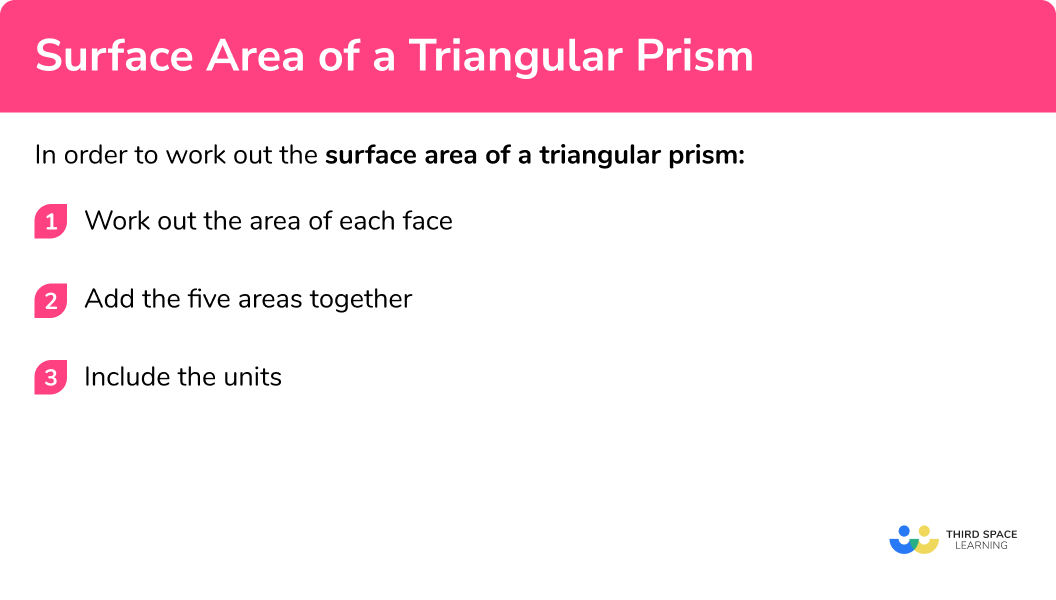# Surface Area Of A Triangular Prism

Here we will learn about the surface area of a triangular prism and how to calculate it.

There are also volume and surface area of a triangular prism worksheets based on Edexcel, AQA and OCR exam questions, along with further guidance on where to go next if you’re still stuck.

## What is the surface area of a triangular prism?

The surface area of a triangular prism is the total area of all of the faces.

To work out the surface area of a triangular prism, we need to work out the area of each face and add them all together.

Lateral faces are all of the faces of an object excluding the top and the base. For a triangular prism the top and the base are triangles and the lateral faces are rectangular sides. The lateral surface area of a triangular prism  is the total area of the rectangular sides

The triangular faces of a triangular prism are congruent (exactly the same) but, unless the triangle is isosceles or equilateral, the rectangles are all different.

E.g.

Since it is an area, surface area is measured in square units (e.g. mm^2, cm^2, m^2 etc).

### What is the surface area of a triangular prism?## How to calculate the surface area of a triangular prism

In order to work out the surface area of a triangular prism:

1. Work out the area of each face.
2. Add the five areas together.
3. Include the units.

### How to calculate the surface area of a triangular prism## Surface area of a triangular prism examples

### Example 1: finding the surface area of a triangular prism with a right triangle

Work out the surface area of the triangular prism

1. Work out the area of each face.

The area of the triangle at the front is \frac{1}{2}\times12\times5 = 30cm^{2}

The back face is the same as the front face so the area of the back is also 30cm^{2} .

The area of the base is 9\times12=108cm^{2}

The area of the left side is 9\times5=45cm^{2}

The area of the top is 9\times13=117cm^{2}

It will make our working clearer if we use a table:

Total surface area: 30 + 30 + 108 + 45 + 117 = 330

3Include the units.

The measurements on the triangular prism are in cm therefore the total surface area of the triangular prism = 330cm^{2} .

### Example 2: surface area of a triangular prism with an isosceles triangle

Work out the surface area of the triangular prism

Total surface area: 28.5 + 28.5 + 84 + 140 + 140 = 421

The measurements on the triangular prism are in mm therefore the total surface area of the triangular prism = 421mm^{2} .

### Example 3: surface area of a right triangular prism

This prism has a triangular base and rectangular sides. We are told the height of the prism is 9cm . We can work out the surface area in exactly the same way, we just adjust the labels we give to each face in our table.

Total surface area: 24 + 24 + 90 + 54 + 72 = 264

The measurements on the triangular prism are in cm therefore the total surface area of the triangular prism = 264cm^{2} .

### Example 4: surface area of a triangular prism

Work out the surface area of the triangular prism

Total surface area: 210 + 210 + 1400 + 480 + 1480 = 3780

The measurements on the triangular prism are in m therefore the total surface area of the triangular prism = 3780m^{2} .

### Example 5: surface area of a triangular prism with different units

Work out the surface area of the triangular prism. Give your answer in mm^{2} .

Some of the measurements here are in cm and some are in mm. Since we have been asked for the answer in mm^{2} , we need to convert all measurements to mm :

1.4cm = 14mm and 2.5cm=25mm .

Total surface area: 56 + 56 + 200 + 350 + 402.5 = 1064.5

The measurements we have used are in mm therefore the total surface area of the triangular prism = 1064.5mm^{2} .

### Example 6: surface area when there is a missing length

Work out the surface area of the triangular prism. Give your answer in cm^{2} .

When calculating the surface area we need the length of each side of the triangle.

We are told the base and height of the triangle and the length of the prism but we don’t have the length of the hypotenuse of the triangle.

To work this out we can use the Pythagorean Theorem a^{2}+b^{2}=c^{2} .

$8^{2}+15^{2} = c^{2} \\ 289 = c^{2} \\ \sqrt{289} = c \\ 17 = c$

Total surface area: 60 + 60 + 72 + 135 + 153 = 480

The measurements we have used are in cm therefore the total surface area of the triangular prism = 480cm^{2} .

### Common misconceptions

• Calculating volume instead of surface area

Volume and surface area are different things – volume tells us the space within the shape whereas surface area is the total area of the faces. To find surface area, work out the area of each face and add them together.

• Thinking all of the rectangles have the same area

Usually all of the rectangle have different areas (unless the triangle is isosceles or equilateral).

• Using the wrong measurements to work out the area of the triangle faces

In surface area questions, we need to know all three side lengths of the triangle however we only need the base and the height to calculate the area of the triangle

Surface area of a triangular prism is part of our series of lessons to support revision on triangular prism. You may find it helpful to start with the main triangular prism lesson for a summary of what to expect, or use the step by step guides below for further detail on individual topics. Other lessons in this series include:

### Practice surface area of a triangular prism questions

1. Work out the surface area of the triangular prism1200 \mathrm{cm}^{2}1040 \mathrm{cm}^{2}920 \mathrm{cm}^{2}1140 \mathrm{cm}^{2}Work out the surface area of each face:

\text{Total surface area }=60+60+160+300+340=920\mathrm{cm}^{2}

2. Work out the surface area of the triangular prism:720 \mathrm{cm}^{2}672 \mathrm{cm}^{2}546 \mathrm{cm}^{2}576 \mathrm{cm}^{2}Work out the surface area of each face:

\text{Total surface area }=48+48+180+150+150=576\mathrm{cm}^{2}

3. Work out the surface area of the triangular prism:82 \mathrm{cm}^{2}94 \mathrm{cm}^{2}36 \mathrm{cm}^{2}66 \mathrm{cm}^{2}Work out the surface area of each face:

\text{Total surface area }=6+6+18+24+30=84\mathrm{cm}^{2}

4. Work out the surface area of the triangular prism.7.007 \mathrm{cm}^{2}14.014 \mathrm{cm}^{2}24.04 \mathrm{cm}^{2}30.89 \mathrm{cm}^{2}Work out the surface area of each face.

\text{Total surface area }=6.37+6.37+2.86+5.39+6.05=27.04\mathrm{cm}^{2}

5. Work out the surface area of the triangular prism. Give your answer in square centimetres.15200 \mathrm{cm}^{2}14400 \mathrm{cm}^{2}2448.8 \mathrm{cm}^{2}96000 \mathrm{cm}^{2}Notice that some of the measurements are in m and some are in cm . Since we are asked to give the answer in square centimetres, we need to convert all the measurements to cm .

0.5m = 50cm and 0.8m=80cm .

Next, Work out the surface area of each face:

\text{Total surface area }=1200+1200+4800+4000+4000=15200 \mathrm{cm}^{2}

6. Work out the surface area of the triangular prism330 \mathrm{m}^{2}936 \mathrm{m}^{2}1560 \mathrm{m}^{2}660 \mathrm{m}^{2}In this question, we are missing the height of the triangle. Since it is a right angled triangle, we can use Pythagoras’ theorem to work out the height:\begin{aligned} a^{2}+b^{2}&=c^{2}\\ h^{2}+12^{2}&=13^{2}\\ h^{2}+144&=169\\ h^{2}&=169-144\\ h^{2}&=25\\ h&=5 \mathrm{m} \end{aligned}

Next, work out the surface area of each face:

\text{Total surface area }=30+30+240+260+100=660 \mathrm{m}^{2}

### Surface area of a triangular prism GCSE questions

1. Work out the surface area of the triangular prism.(3 marks)

\frac{1}{2} \times 0.3 \times 0.4 = 0.06

(1)

1 \times 0.3=0.1, ~1 \times 0.4 – 0.4,~ 1 \times 0.5 = 0.5

(1)

0.06+0.06+0.3+0.3+0.5=1.32 \mathrm{m}^{2}

(1)

2. A packaging company wants to minimise the amount of packaging they use. Which of these shapes should they choose to make their packaging? Show how you decide.(5 marks)

Prism A: Surface area = 24+24+90+120+150

(1)

\text{Surface area }=408 \mathrm{cm}^{2}

(1)

Prism B: Surface area = 25+25+140+70+156.8

(1)

\text{Surface area }=416.8 \mathrm{cm}^{2}

(1)

They should use shape A

(1)

3. A packaging box is made in the following shapeThe material used to make the box costs 0.15p per \mathrm{cm}^{2} to produce. How much would it cost to make 100 boxes? Give your answer in pounds.

(5 marks)

Surface area = 12+12+88+55+55

(1)

\text{Surface area }=222 \mathrm{cm}^{2}

(1)

222 \times 0.15 = 33.3

(1)

33.3 \times 100=3330 \text{p}

(1)

3330p=£33.30

(1)

## Learning checklist

You have now learned how to:

• Calculate the surface area of a triangular prism
• Use the properties of faces, surfaces, edges and vertices of cubes and cuboids to solve problems in 3-D

## Still stuck?

Prepare your KS4 students for maths GCSEs success with Third Space Learning. Weekly online one to one GCSE maths revision lessons delivered by expert maths tutors.

Find out more about our GCSE maths tuition programme.